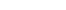Loading... Please wait...

# MAT 230 MAT230 Module 3 Homework (SNHU)

Price:
\$14.99

Click the button below to add the MAT 230 MAT230 Module 3 Homework (SNHU) to your wish list.

SHARE

## Product Description

MAT 230 MAT230 Module 3 Homework (SNHU)

Appendix A Homework

1) The following function written in pseudocode accepts INCOMEas a variable and outputs the TAX corresponding to that income.

2) Suppose that the array X consists of real numbers X, X, the array Y consists of the real numbers Y, Y, and the array Z consists of the real numbers Z, Z. What does the following algorithm compute?

3) Consider the following algorithm; assume N to be a positive integer.

Section 1.4 Homework

1) Write m as qn+r, with 0 < r < n for parts a) through c).

2) Write the integer as a product of powers of primes.

3) Find the greatest common divisor d of the integers 58 and 124, and write d as s(58) + t(124). Write out all of the steps.

4) Use the fact that GCD(a,b)•LCM(a,b)=ab to compute the least common multiple of 58 and 124, LCM(58,124).

5) If f is the mod-5 function, compute each of the following:

6) Use Bacon’s code to create a dummy message for ABANDON. For the sake of simplicity, use bold font for 0 and regular font for 1.

Section 2.4 Homework

1) Prove that

Here is a general outline you can use.

Basis step. Prove P(1)

Induction step.

Write out P(k) by replacing “n” with “k” in the original equation.

Now, we must look at P(k+1). To do this, modify your P(k) expression from part 2a by adding the “k+1”st term to the left hand side and replace “k” with “k+1” on the right hand side. This is what we need to show.

Using the assumption that P(k) is true, replace the “1 through k” portion on the left hand side of the P(k+1) equation you wrote in part 2b.

If necessary, multiply away any constant denominators in the new P(k+1) formulation.

Multiply out the left-hand side and the right-hand side to establish the equality.

## Product Reviews

This product hasn't received any reviews yet. Be the first to review this product!

• \$4.00
• \$99.99
• \$10.00
• \$14.99
• \$14.99

• \$4.00
• \$99.99
• \$10.00
• \$14.99
• \$14.99

## Related Products

• \$14.99• \$8.99• \$12.99• \$12.99• \$12.99